Students can go through AP SSC 10th Class Maths Notes Chapter 8 Similar Triangles to understand and remember the concepts easily.

## AP State Syllabus SSC 10th Class Maths Notes Chapter 8 Similar Triangles

→ The geometrical figures which have the same shape but are not necessarily of the same size are called similar figures.

→ The heights and distances of distant objects can be found using the principles of similar figures.

→ Two polygons with same number of sides are said to be similar if their corresponding angles are equal and their corresponding sides are in proportion.

→ A polygon in which all sides and all its angles are equal is called a regular polygon. Eg.: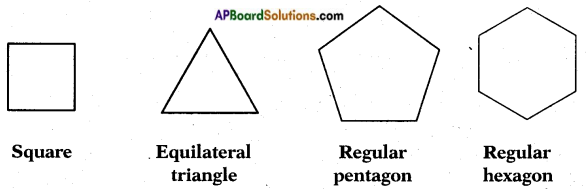→ The ratio of the corresponding sides is referred to as scale factor or representative factor.

→ All squares are similar.

→ All circles are similar.

→ All equilateral triangles are similar.

→ Two congruent figures are similar but two similar figures need not be congruent.

→ A square ABCD and a rectangle PQRS are of equal corresponding angles, but their corre¬sponding sides are not in proportion.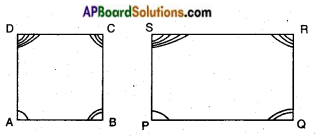∴ The square ABCD and the rectangle PQRS are not similar.

→ The corresponding sides of a square ABCD and a rhombus PQRS are equal but their corresponding angles are not equal. So they are not similar.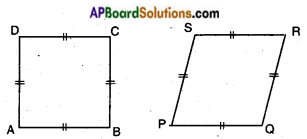→ If a line is drawn parallel to one side of a triangle intersecting the other two sides at two distinct points then the other two sides are divided in the same ratio.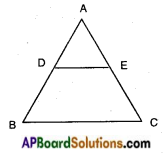In △ABC; DE // BC then $$\frac{AD}{DB}$$ = $$\frac{AE}{EC}$$.
This is called Basic proportionality theorem (or) Thale’s theorem.→ If a line divides any two sides of a triangle in the same ratio, then the line must be parallel to the third side.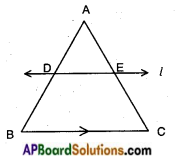In △ABC, a line ‘l’ intersecting AB in D and AC in E
such that $$\frac{AD}{DB}$$ = $$\frac{AE}{EC}$$ then l // BC.
This is converse of Thale’s theorem.

→ Two triangles are similar, if
i) their corresponding angles are equal.
ii) their corresponding sides are in the same ratio.

→ If in two triangles, corresponding angles are equal, then their corresponding sides are in the same ratio or proportional and hence the two triangles are similar.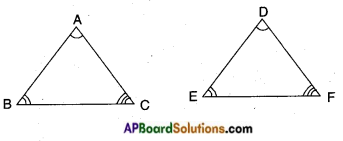In △ABC, △DEF
∠A = ∠D
∠B = ∠E
∠C = ∠F
⇒ $$\frac{AB}{DE}$$ = $$\frac{BC}{EF}$$ = $$\frac{AE}{DF}$$
∴ △ABC ~ △DEF (A.A.A)→ If in two triangles, sides of one triangle are proportional to the sides of other triangle, then their corresponding angles are equal and hence the two triangles are similar.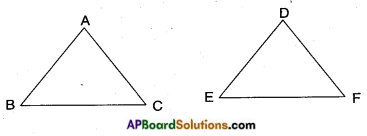In △ABC, △DEF
if $$\frac{AB}{DE}$$ = $$\frac{BC}{EF}$$ = $$\frac{AE}{DF}$$
⇒ ∠A = ∠D
∠B = ∠E
∠C = ∠F
Hence, △ABC ~ △DEF (S.S.S)

→ If two angles of a triangle are equal to two corresponding angles of another triangle then the two triangles are similar.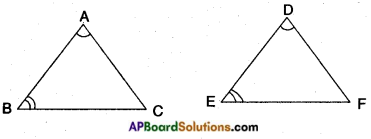In △ABC, △DEF
if ∠A = ∠D
∠B = ∠E
⇒ ∠C = ∠F (By Angle Sum property)
∴ △ABC ~ △DEF (A.A)

→ If one angle of a triangle is equal to one angle of other triangle and the sides including these angles are proportional, then the two triangles are similar.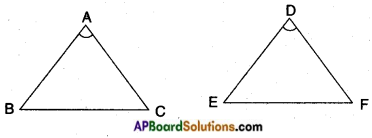In △ABC, △DEF if ∠A = ∠D, and
$$\frac{AB}{DE}$$ = $$\frac{AC}{DF}$$
⇒ △ABC ~ △DEF (S.A.S)→ The ratio of areas of two similar triangles is equal to the ratio corresponding sides.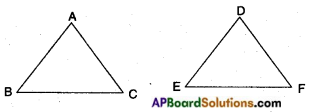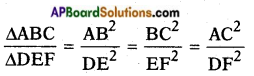→ If a perpendicular is drawn from the vertex, containing the right angle of a right angled – triangle to the hypotenuse, then the triangles on each side of perpendicular are similar to one another and to the original triangle. Also the square of the perpendicular is equal to the product of the lengths of the two parts of the hypotenuse.
In △ABC, ∠B = 90°
BD ⊥ AC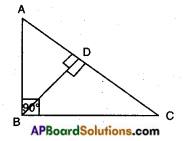Then △ADB ~ △BDC ~ △ABC and

→ Pythagoras theorem: In a right angled triangle, the square of hypotenuse is equal to the sum of the squares of other two sides.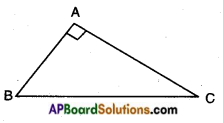In △ABC; ∠A = 90°
AB2 + AC2 = BC2

→ In a triangle, if square of one side is equal to sum of squares of the other two sides, then the angle opposite to the first side is right angle.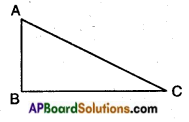In △ABC, if
AC2 = AB2 + BC2 then ∠B = 90°
This is converse of Pythagoras theorem.→ Baudhayan Theorem (about 800 BC):
The diagonal of a rectangle produces itself the same area as produced by its both sides (i.e., length and breadth).
In rectangle ABCD,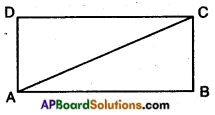area produced by the diagonal AC = AC • AC
= AC2
area produced by the length = AB • BA = AB
area produced by the breadth = BC • CB = BC2
Hence, AC2 = AB2 + BC2.

→ A sentence which is either true or false but not both is called a simple statement.

→ A statement formed by combining two or more simple statements is called a compound statement.

→ A compound statement of the form “If …… then ……” is called a Conditional or Implication.

→ A statement obtained by modifying the given statement by ‘NOT’ is called its negation.